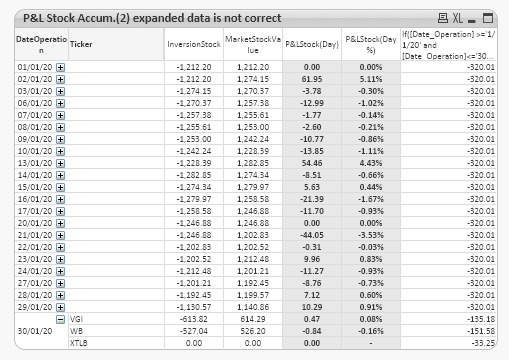# New to QlikView

Discussion board where members can get started with QlikView.

Announcements
Leverage your QlikView investment to modernize BI – see how! Join Group
cancel
Showing results for
Did you mean:
HighlightedContributor III

## Select an specific date value by dimension in set analysis when using a date range

Hi,

In one column I'm using a set analysis expression to get all sum(values) between a date range.

Example: sum({<[Date_Operation] = {">=\$(=date(vStartDate))<=\$(=date(vEndDate))"}>} Aggregate_Diary_PL_Stock_Accum)

With this expression there's no problem and I can get the corresponding value for each dimension in each date.

In another column I'm using set analysis to get the sum(values) in a specific date "vStartDate-1"

Example: sum(total {<[Date_Operation] = {"\$(=date(vStartDate-1))"}>} Aggregate_Diary_PL_Stock_Accum)

The problem that I have with that expression is that I need to use 'total' to get the sum(values) correctly, but then I can't get the value for each dimension.

I've already tried total <dimension> but it doesn't work... it only works when using vEndDate intead of vStartDate-1, that's why I suppose that the problem is the Date_Operation of each row.

So, In the second expression I would have to avoid the Date_Operation field, but I can't manage it.

Any help would be appreciated.

Labels (3)

• ### Totals not working

1 Solution

Accepted Solutions
HighlightedMVP

## Re: Select an specific date value by dimension in set analysis when using a date range

Hi have worked for one expression and left some for you. 😋 . The main change in expression is use of dimensionality() and replacing set analysis with IF.

``````If([Date_Operation] >='\$(=date(vStartDate))' and [Date_Operation]<='\$(=date(vEndDate))',
If( Dimensionality()=2
,Aggr( sum(TOTAL <Ticker> If([Date_Operation] ='\$(=date(vStartDate-1))', Aggregate_Diary_PL_Stock_Accum)), Date_Operation,Ticker)
,sum(TOTAL {<[Date_Operation] = {"\$(=date(vStartDate-1))"}>} Aggregate_Diary_PL_Stock_Accum)
)
,
0
)   ``````13 Replies
HighlightedContributor III

## Re: Select an specific date value by dimension in set analysis when using a date range

Sorry to bother you @sunny_talwar, but there is no reply to my post and I'm sure that you have the solution.

Best Regards

Wale

HighlightedMVP

## Re: Select an specific date value by dimension in set analysis when using a date range

I guess you are on the right path, Total is required to be used here. Could you explain with a sample or screenshot what you are getting using total identifier and what you are looking for?

HighlightedContributor III

## Re: Select an specific date value by dimension in set analysis when using a date range

Hi @tresesco ,

Collapsed Data

In that case 'P&L StockAccum.' calculation is correct. 4,343.98 is the correct total (all tickers) value for 'date(vStartDate-1)'

Expanded Data (Example 30 January)

In that case 'P&L StockAccum.' calculation is incorrect. As you can see, for each ticker I'm assigning the 'date(vStartDate-1)' total (all tickers) value and not the ticker one.

I don't know if I'm explaining myself well.

HighlightedMVP

## Re: Select an specific date value by dimension in set analysis when using a date range

A sample qv file would have been of more help in knowing what expression, dimensions...you are using. Could you share one? Else, help explaining the dimensions you are using in chart (possibly pivot) and exact expression you are using.

Assuming you are expecting different number for every tickers, in other words, respect the ticker dimension.

HighlightedContributor III

## Re: Select an specific date value by dimension in set analysis when using a date range

Sorry @tresesco ,

But I can't share the qv file (sensitive information in it). Let me prepare a sample. I'll attach it as soon as possible.

Thanks

HighlightedContributor III

## Re: Select an specific date value by dimension in set analysis when using a date range

Hi @tresesco ,

Attached you have a qv example with its dataset

Thank you very much

Wale

HighlightedContributor III

## Re: Select an specific date value by dimension in set analysis when using a date range

sorry @tresesco

This qv file will be clerarer.

HighlightedMVP

## Re: Select an specific date value by dimension in set analysis when using a date range

What if you try :

sum( total <Ticker>  {<[Date_Operation] = {"\$(=date(vStartDate-1))"}>} Aggregate_Diary_PL_Stock_Accum)

HighlightedContributor III

## Re: Select an specific date value by dimension in set analysis when using a date range

Hi @tresesco,

That was one of the first options that I tried... but all values were converted into "0"